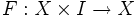# Isotopy

Let$X$ be a topological space and$f,g$ be homeomorphisms from$X$ to itself. An isotopy from$f$ to$g$ is a homotopy$F:X \times I \to X$ that starts at$f$, ends at$g$, and such that for any fixed$t$, the map$x \mapsto F(x,t)$ is a homeomorphism.